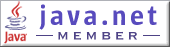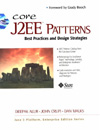Core J2EE Pattern Catalog
 Last Updated: November 2, 2003 10:24 PMsince 02/16/2002

This page provides the sample code for the First edition. We are in the process of updating this site to provide the sample code for the Second Edition. We thank you for your patience.

Sample Code for 1st EditionChapter 3

 Example 3.1 Example 3.2 Example 3.3 Example 3.4 Example 3.5 Example 3.6

Chapter 5

 Example 5.1 Example 5.2 Example 5.3 Example 5.4 Example 5.5 Example 5.6 Example 5.7 Example 5.8 Example 5.9 Example 5.10 Example 5.11

Chapter 7

 Example 7.1 Example 7.2 Example 7.3 Example 7.4 Example 7.5 Example 7.6 Example 7.7 Example 7.8 Example 7.9 Example 7.10 Example 7.11 Example 7.12 Example 7.13 Example 7.14 Example 7.15 Example 7.16 Example 7.17 Example 7.18 Example 7.19 Example 7.20 Example 7.21 Example 7.22 Example 7.23 Example 7.24 Example 7.25 Example 7.26 Example 7.27 Example 7.28 Example 7.29 Example 7.30 Example 7.31 Example 7.32 Example 7.33 Example 7.34 Example 7.35

Chapter 8

 Example 8.1 Example 8.2 Example 8.3 Example 8.4 Example 8.5 Example 8.6 Example 8.7 Example 8.8 Example 8.9 Example 8.10 Example 8.11 Example 8.12 Example 8.13 Example 8.14 Example 8.15 Example 8.16 Example 8.17 Example 8.18 Example 8.19 Example 8.20 Example 8.21 Example 8.22 Example 8.23 Example 8.24 Example 8.25 Example 8.26 Example 8.27 Example 8.28 Example 8.29 Example 8.30 Example 8.31 Example 8.32 Example 8.33 Example 8.34 Example 8.35

Chapter 9

 Example 9.1 Example 9.2 Example 9.3 Example 9.4 Example 9.5 Example 9.6 Example 9.7 Example 9.8 Example 9.9